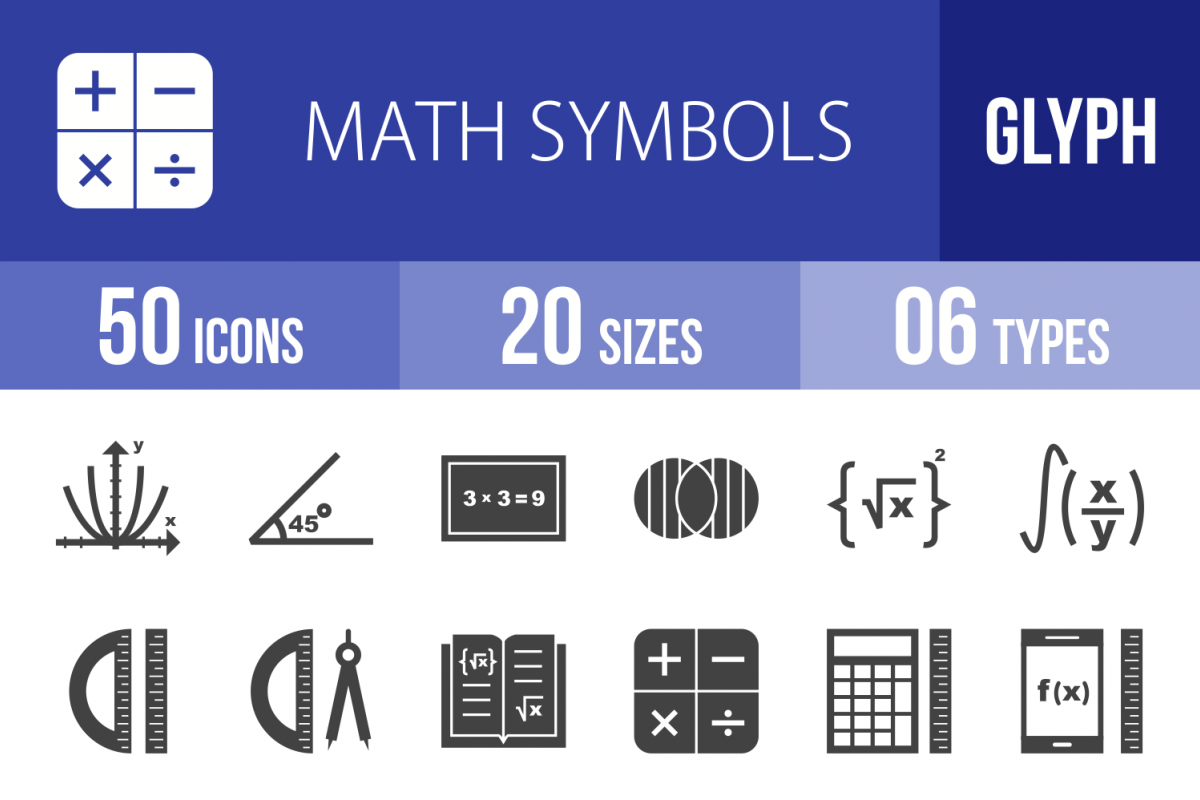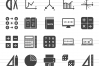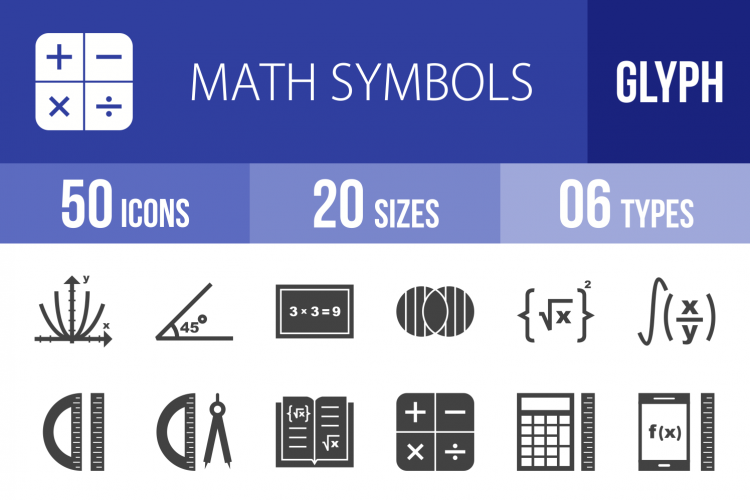# 50 Math Symbols Glyph Icons2 Favorites

**50 Math Symbols Glyph Icons**

Suitable for: Mobile Apps, Websites, Print, Presentation, Illustration, Templates

Features:
- Ready to use for all devices and platforms
- 6 Different formats: AI, CDR, EPS, JPG, PNG, SVG
- Designed using unigrid system
- Each Math Symbols icon is designed for maximum usability
- 100% vector icons
- Easy to edit and scale
- 20 PNG Sizes

50 Icons:

- Algebra
- Angle
- Arithmetic
- Bell Shaped Graph
- Calculator
- Circle
- Compass
- Cosine Graph
- Cube
- Cylinder
- Discrete Math
- Drawing Graphs
- Formula I
- Formula II
- Geometry Tools I
- Geometry Tools II
- Graph I
- Graph II
- Integral
- Linear Function
- Logic
- Math Book I
- Math Book II
- Math in Computer
- Math Symbols I
- Math Symbols II
- Measurement Calculation
- Number Theory
- Online Calculation
- Online Formula
- Pencil and Set Square
- PIe Chart
- Print Math Sheet
- Probability
- Ruler and Set Square
- Set Square and Graph
- Solve Function
- Solve Problem
- Solving Formula
- Statistics
- Stereometry
- Studying Geometry
- Studying Math on Mobile
- Subsets
- Equal to Symbol
- Infinity Symbol
- Summation Symbol
- Percentage Symbol
- Square Root Symbol
- Value of Pi

20 PNG File Sizes:
1. 16×16
2. 24×24
3. 29×29
4. 32×32
5. 40×40
6. 48×48
7. 58×58
8. 64×64
9. 72×72
10. 76×76
11. 80×80
12. 96×96
13. 120×120
14. 128×128
15. 144×144
16. 152×152
17. 192×192
18. 256×256
19. 512×512
20. 1024×1024

Hope you like our iconset!

2
1
0
0
0

### Product Information

Release Date: 25th January '18

### How to use

Introducing Design School – our jam packed platform with its own mini search engine! Design School was made to help you learn all there is to know about fonts, graphics, templates, designs, file types, different software programs, Frequently Asked Questions and much much more.

### Similar Design Products to 50 Math Symbols Glyph Icons#### 50 Math Symbols Glyph Icons

By Iconbunny